Question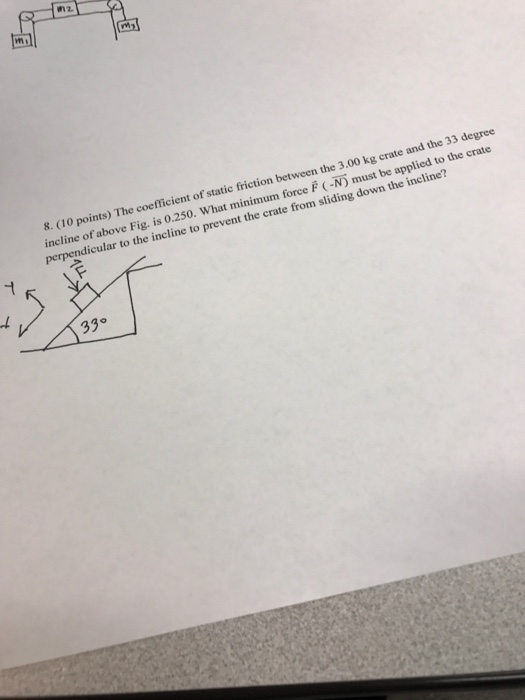Normal force

N = mg cos33 + F

for minimum value of F we have

u N = mg sin 33

substituting values we get

F = 39.39 N

#### Earn Coins

Coins can be redeemed for fabulous gifts.

Similar Homework Help Questions
• ### The coefficient of static friction between the m = 3.85-kg crate and the 35.0° incline of...

The coefficient of static friction between the m = 3.85-kg crate and the 35.0° incline of the figure below is 0.325. What minimum force F must be applied to the crate perpendicular to the incline to prevent the crate from sliding down the incline? N

• ### The coefficient of static friction between them.3.20-kg rate and the 3.0 incline of the figure below...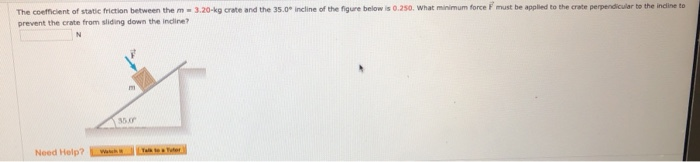The coefficient of static friction between them.3.20-kg rate and the 3.0 incline of the figure below is 0.250. What minimum force must be polied to the crate perpendicular to the indireto prevent the rate from sliding down the indine? Need Help?

• ### A 5 kg block rests on a 30 degree incline. The coefficient of static friction between...

A 5 kg block rests on a 30 degree incline. The coefficient of static friction between the block and the incline is .20. How large a horizontal force must push on the block if the block is to be on the verge of sliding A) up the incline and B) down an incline Answer: A) 43 N B) 16.6 N What would be the work to get me to this answer?

• ### A large crate is at rest on a horizontal floor. The coefficient of static friction between...

A large crate is at rest on a horizontal floor. The coefficient of static friction between the crate and the floor is 0.500. A force F⃗  is applied to the crate in a direction 30.0∘ above the horizontal. The minimum value of F required to get the crate to start sliding is 410 N. Part A What is the mass of the crate? Express your answer with the appropriate units.

• ### physics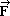The coefficient of static friction between the m =3.20 kg crate and the 35.0°incline of Figure P4.41 is 0.340. Whatminimum force must be applied to the crate perpendicular to the inclineto prevent the crate from sliding down theincline? NFigure P4.41

• ### Figure 5 shows a crate has a mass of 50 kg and the coefficient of static...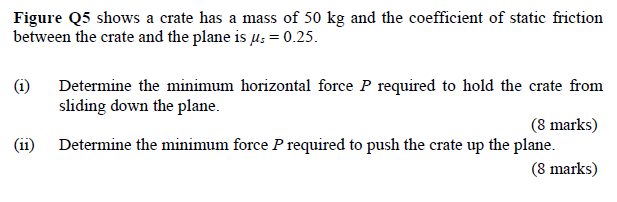Figure 5 shows a crate has a mass of 50 kg and the coefficient of static friction between the crate and the plane is us = 0.25. (1) Determine the minimum horizontal force P required to hold the crate from sliding down the plane. (8 marks) Determine the minimum force P required to push the crate up the plane. (8 marks) (11) P 30° Figure Q5

• ### A 5.0 kg crate is resting on a horizontal plank. The coefficient of static friction is...

A 5.0 kg crate is resting on a horizontal plank. The coefficient of static friction is 0.50 and the coefficient of kinetic friction is 0.40. One end of the plank is raised so the plank makes an angle of 30° with the horizontal. Find the minimum force that can be applied parallel to the plane to hold the crate at rest. a. 4.49N b. 0 c. 2.29N d. 3.29N e. 5.59N

• ### Please help The mass of block in the figure is 9 kg. The coefficient of static...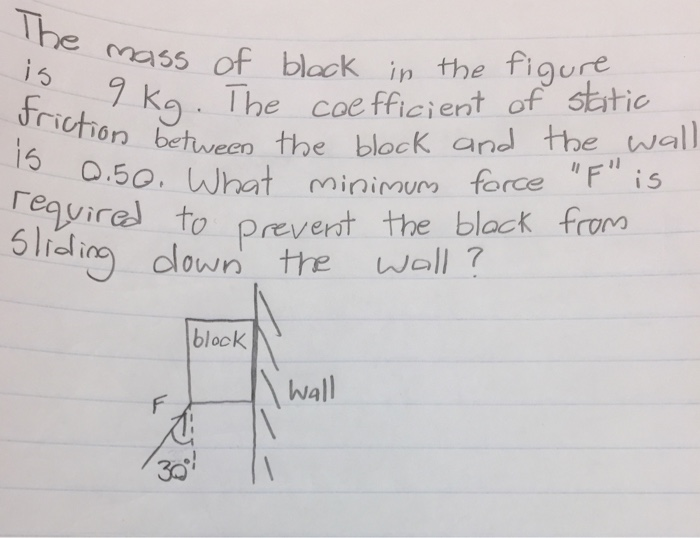Please help The mass of block in the figure is 9 kg. The coefficient of static friction between the block and the wall is 0.50. What minimum force "F" is required to prevent the block from sliding down the wall?

• ### Friction coefficient of friction support force, sliding or static coefficient F and angle in polar format...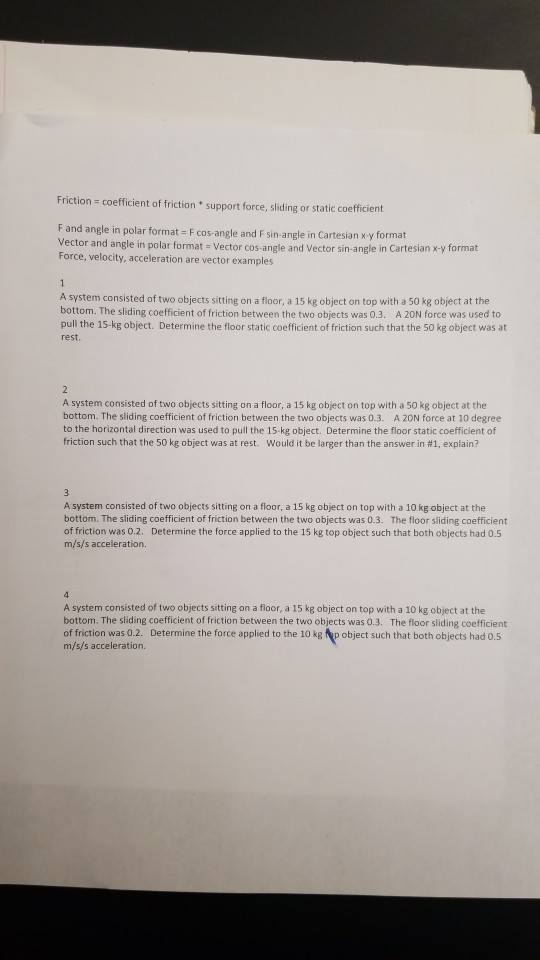Friction coefficient of friction support force, sliding or static coefficient F and angle in polar format F cos-angle and F sin-angle in Cartesian x y format Vector and angle in polar format - Vector cos-angle and Vector sin-angle in Cartesian x-y format Force, velocity, acceleration are vector examples A system consisted of two objects sitting on a floor, a 15 kg object on top with a 50 kg object at the bottom. The sliding coefficient of friction between the two...

• ### A 313-kg crate rests on a surface that is inclined above the horizontal at an angle...

A 313-kg crate rests on a surface that is inclined above the horizontal at an angle of 17.0°. A horizontal force (magnitude = 414 N and parallel to the ground, not the incline) is required to start the crate moving down the incline. What is the coefficient of static friction between the crate and the incline?